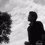# Interesting combo.

For $\frac 1{\omega_n} =\prod_{k=1}^{n}\cot^{2}\left(\dfrac{k\pi}{2n+1}\right)\left(\sum_{k=0}^{2n}\cot\left(x+\frac{k\pi}{2n+1}\right)\right)$

Find$\Omega =\lim_{n\to\infty}\left(\lim_{x\to\frac{\pi}{4n+2}}\left(\dfrac{\tan x}{\tan\left(\frac{\pi}{4n+2}\right)}\right)^{\omega_n}\right)$ This is the proposal by teachers Marian Ursărescu and Florică Anastase, Romania and published by Romanian Mathematical Magazine.

Initially the answer of proposers agreed with me but later we had a slight different conclusions. As the per the proposers they arrived to $\frac{1}{\sqrt[\pi]{e^4}}$.

If the answer of proposers is correct then probably I think I had done mistakes while evaluating the limits. I even tried on wolfram alpha it agrees with me ( doesn't provide the closed form but it approximates to my closed form) . Can anybody help me to correct my mistakes ? Thank you ! :)

Solution Here i wish to share my solution.

Firstly we recall Euler's formula$e^{imx} = (\cos mx + i\sin mx)= (\cos x+ i\sin x)^m$ and further solving gives $\frac{\cos mx +i\sin mx}{\sin^m x}=$$\displaystyle \sum_{r=0}^m { m\choose r} \cot^{m-r}x i^{r}= \displaystyle \sum_{r=0}^{\lceil \frac{m}{2}\rceil+ l }(-1)^{r}{ m\choose 2r} \cot^{m-2r}x+i\sum_{r=1}^{\lceil \frac{m}{2}\rceil}{ m\choose 2r-1}(-1)^{r} \cot^{m-2r+1}x$ where $l=1,0$ if $m$ is even and odd respectively. We note that latter sum is the imaginary part and hence equating we get $\sum_{r=1}^{\lceil \frac{m}{2}\rceil}{ m\choose 2r-1} \cot^{m-2r+1}x=\frac{\sin mx}{\sin^{m}x}$ on setting $m= 2n+1, \; x= \frac{k\pi}{m}$ and further expansion we get ${ 2n+1 \choose 1} \cot^{2n} \frac{k\pi}{2n+1} - { 2n+1 \choose 3} \cot^{2n-2}\frac{k\pi}{2n+1} +\cdots + { 2n+1 \choose 2n+1} =0$ as the polynomial equation is of even degree and hence by Vieta's formula we have $\prod_{k=1}^{n} \cot^2\left(\frac{k\pi}{2n+1}\right)=(-1)^{2n}\frac{{ 2n+1\choose 2n+1}}{{ 2n+1\choose 1}}=\frac{1}{2n+1}$ secondly we use the identity $\displaystyle\prod_{k=0}^{n-1} \sin\left(x+\frac{k\pi}{n}\right) =\frac{\sin nx}{2^{n-1} }$, taking $\log$ on both side and on differentiating with respect to $x$, we get that, ie $\frac{d}{dx}\log\left(\displaystyle\prod_{k=0}^{n-1} \sin\left(x+\frac{k\pi}{n}\right)\right) =\frac{d}{dx}\log\left(\frac{\sin nx}{2^{n-1}}\right)\\ \Rightarrow \displaystyle \sum_{k=0}^{n-1} \cot\left(x+\frac{k\pi}{n}\right) =n\cot(nx)$. Replacing $n$ by $2n+1$ we yield $\displaystyle \sum_{k=0}^{2n} \cot\left(x+\frac{k\pi}{2n+1}\right) =(2n+1)\cot((2n+1)x)$and we deduce that $\omega_n = \left(\frac{(2n+1)\cot ((2n+1)x)}{2n+1}\right)^{-1} = \tan ((2n+1)x)$.

Call $L(n)= \displaystyle\lim_{x\to\frac{\pi}{4n+2}}\left(\frac{\tan x}{\tan \beta} \right)^{\omega_n}$. As we can observe that we have $1^{\infty}$ limit form. Since as soon as $x\to\frac{1}{4n+2}$,function $w_n\to\infty$ and $\frac{\tan x}{\tan\beta}\to 1$ with $\beta =\frac{\pi}{4n+2}$. So we can either make the direct use the formula for $1^{\infty}$ or without of it too. ie

$\displaystyle\lim_{x\to\beta}\left(\frac{\tan x}{\tan \beta}\right)^{\omega_n}=\displaystyle\lim_{x\to\beta}\left(1+\frac{\tan x}{\tan \beta}-1\right)^{\omega_n}= \displaystyle\lim_{x\to\beta}\left(1+\frac{1}{\frac{\tan x}{\tan \beta}-1}\right)^{\omega_n} \\= \exp\left(\displaystyle\lim_{x\to\beta }\left(\frac{\tan x}{\tan \beta }-1\right)\tan((2n+1)x)\right)=\exp\left(\displaystyle\lim_{x\to\beta }\left(\frac{\tan x-\tan\beta}{\tan\beta \cot ((2n+1)x)}\right)\right)$. Here we have the limit of the form $\frac{0}{0}$ so we use L-hopital's rule to get $\exp\left(\displaystyle \lim_{x\to\beta}\frac{\sec^2 x}{-(2n+1)\csc^2((2n+1)x)\tan\beta}\right)=\exp\left(\frac{\sec \beta}{-\sin\beta (2n+1)}\right)$. And therefore, $\lim_{n\to\infty} L(n)=\exp\left(-\displaystyle \lim_{n\to\infty} \frac{\frac{2\pi}{\pi(4n+2)}}{\tan \frac{\pi}{4n+2}}\right)=\exp\left(-\frac{2}{\pi}\displaystyle \lim_{n\to\infty} \frac{\frac{\pi}{(4n+2)}}{\tan \frac{\pi}{4n+2}}\right) =e^{-\frac{2}{\pi}}=\frac{1}{\sqrt[\pi]{e^{2}}}$Note by Naren Bhandari
1 year, 3 months ago

This discussion board is a place to discuss our Daily Challenges and the math and science related to those challenges. Explanations are more than just a solution — they should explain the steps and thinking strategies that you used to obtain the solution. Comments should further the discussion of math and science.

When posting on Brilliant:

• Use the emojis to react to an explanation, whether you're congratulating a job well done , or just really confused .
• Ask specific questions about the challenge or the steps in somebody's explanation. Well-posed questions can add a lot to the discussion, but posting "I don't understand!" doesn't help anyone.
• Try to contribute something new to the discussion, whether it is an extension, generalization or other idea related to the challenge.
• Stay on topic — we're all here to learn more about math and science, not to hear about your favorite get-rich-quick scheme or current world events.

MarkdownAppears as
*italics* or _italics_ italics
**bold** or __bold__ bold
- bulleted- list
• bulleted
• list
1. numbered2. list
1. numbered
2. list
Note: you must add a full line of space before and after lists for them to show up correctly
paragraph 1paragraph 2

paragraph 1

paragraph 2

[example link](https://brilliant.org)example link
> This is a quote
This is a quote
    # I indented these lines
# 4 spaces, and now they show
# up as a code block.

print "hello world"
# I indented these lines
# 4 spaces, and now they show
# up as a code block.

print "hello world"
MathAppears as
Remember to wrap math in $$ ... $$ or $ ... $ to ensure proper formatting.
2 \times 3 $2 \times 3$
2^{34} $2^{34}$
a_{i-1} $a_{i-1}$
\frac{2}{3} $\frac{2}{3}$
\sqrt{2} $\sqrt{2}$
\sum_{i=1}^3 $\sum_{i=1}^3$
\sin \theta $\sin \theta$
\boxed{123} $\boxed{123}$

## Comments

Sort by:

Top Newest

@Mark Hennings Sir, can you help me?
Thank you !! :)

- 1 year, 3 months ago

Log in to reply

I tend to agree with you The inner limit is $\lim_{x \to \beta} \left(\frac{\tan x}{\tan \beta}\right)^{\tan\big(\tfrac{\pi x}{2\beta}\big)} \; = \; \lim_{y \to 0}\left(\frac{\tan(\beta-y)}{\tan\beta}\right)^{\cot\big(\tfrac{\pi y}{2\beta}\big)} \; = \; \lim_{y \to 0}F(y)$ where $\beta = \tfrac{\pi}{4n+2}$, so that \begin{aligned} \lim_{y \to 0}\ln F(y) & = \; \lim_{y \to0}\frac{\ln\left(\frac{\tan(\beta-y)}{\tan\beta}\right)}{\tan \tfrac{\pi y}{2\beta}} \; = \; \lim_{y \to 0} \frac{-\frac{\sec^2y}{\tan \beta - \tan y} - \frac{\tan\beta \sec^2y}{1 + \tan\beta\tan y}}{\frac{\pi}{2\beta}\sec^2\tfrac{\pi y}{2\beta}} \\ & = \; -\frac{2\beta}{\pi}(\tan\beta + \cot\beta) \; = \; - \frac{4\beta}{\pi \sin2\beta} \end{aligned} which in turn tends to $-\tfrac{2}{\pi}$ as $n \to \infty$, so that $\beta \to 0$. Exponentiating gives the limit $\frac{1}{\sqrt[\pi]{e^2}}$.

I have not looked at the original question. You have transcribed it correctly?

- 1 year, 3 months ago

Log in to reply

Yes, I have it correctly, Sir. I don't know how to attach the photo of it here in comment section so I'm here providing the link of it Original problem as I got this problem on Facebook wall of proposer. Please do check once. Thank your very much for your response. :)

- 1 year, 3 months ago

Log in to reply

×

Problem Loading...

Note Loading...

Set Loading...